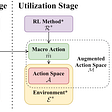# GAN — LSGAN (How to be a good helper?)

The GAN discriminator distinguishes real images from the generated one. In practice, the discriminator usually performs reasonably well. But as a critic for the generator, how helpful is it? As part of the GAN series, we look into LSGAN (Least squares GAN) on how a cost function may improve the GAN training.

When the discriminator is optimal, the objective function of the generator becomes (proof)

Below, we plot the JS-divergence for p (a Gaussian distribution with mean=0) with different Gaussian distributions q with means ranging from 0 to 30.

When pr and pg (the data distributions for the real and the generated images) are far apart, the gradient for their JS-divergence vanishes and the gradient descent will not be effective.

In short, the discriminator performs well but it is a lousy critic for the generator.

Least Squares GAN (LSGAN) is designed to help the generator better. Mathematically, let’s define the new objective functions to be

where a and b are the target discriminator labels for the generated images and the real images, and c is target generator labels for the generated images. Now, the question is what is the value for a, b and c so pg will converge to pr during training. We add an extra term to the generator equation. Since D(x)-c is not related to G, the optimal point for the equation remains the same.

The optimal discriminator with a fixed G will be:

Without proof, we can transform the cost function for the generator to:

This is a Pearson χ2 divergence. Its significant is we can get a smoother gradient everywhere. When pg and pd are different, the gradient does not vanish, and the solution converges as pg approaches pd. By picking a = −1, b = 1, and c = 0, we get

LSGAN makes another proposal to use c=b=1 and a=0.

Intuitively, LSGAN wants the target discriminator label for real images to be 1 and generated images to be 0. And for the generator, it wants the target label for generated images to be 1.

For your reference, this is the original GAN’s

Experiments in the LSGAN paper demonstrate similar performance for both equations. For the experiments in the paper, the second set of equations are used.

# Network design

The following is the network design for the generator and the discriminator.

For dataset provided with labels, we can use the CGAN to generate the images. In particular, the quality will improve if there are many object classes. Here is the network architect to use CGAN and LSGAN together.

# Results

--

--

--

## More from Jonathan Hui

Deep Learning

Love podcasts or audiobooks? Learn on the go with our new app.

## A Glimpse Into The Future of Quantum Machine Learning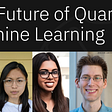## Tensorflow2.0 HelloWorld using Google Colab## Hardware Accelerated Search For Drug Discovery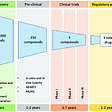## Machine Learning Optimization techniques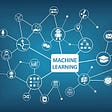## Intro to Markov Chains## 4 Google Colabs Tips and Tricks for Machine Learning## Natural Language Processing for Beginners: A simple Illustration in Python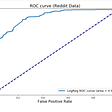Deep Learning

## Review: Sparse Transformer## RL — Model-Based Learning with Raw Videos## Meta Learning — A Overly Simple Overview## [ACM TELO 2021 / NeurIPS 2020 Works] Reusability and Transferability of Macro Actions for…